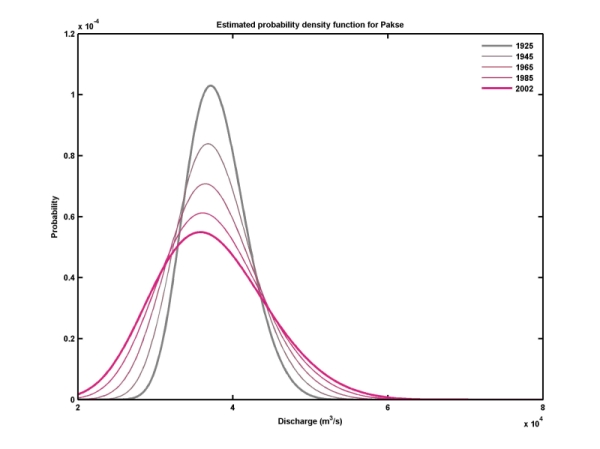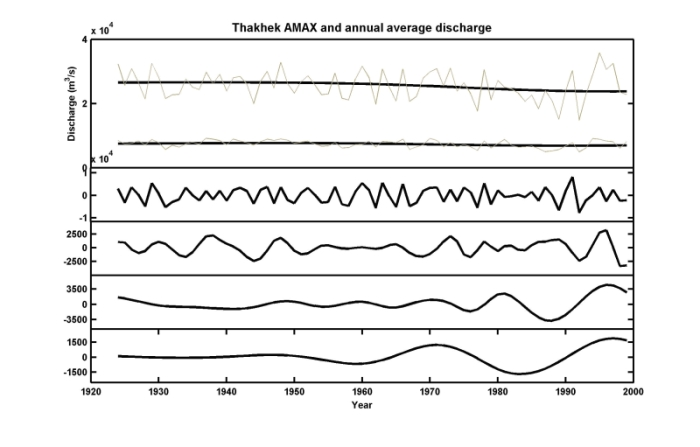Fontsize: [-] Text [+]

# Hydrology of the Mekong Basin – non-stationary time series analysis

At a first step in time series analysis efforts to identify average trends in annual maximum discharge and 90-day annual minimum discharge in the gauges Vientiane, Thakhek, Pakse and Kratie were undertaken. In a further step the use of non-linear techniques for assessment of variability along time in the dataset, considered a fundamental aspect to characterize hydrological change in the catchment. Adding to the results then presented, and in order to validate those, other techniques were now explored. In order to assess the results regarding variability, the wavelet power spectrum was used. Through this technique it is possible to locate significant changes in amplitude of the signal both in time and frequency. An example of the results obtained is Figure 1, where the wavelet power spectrum is plotted for Pakse (Laos), showing a strong change in amplitude in the last quarter of the century. This is a common feature to both Thakhek (Laos) and Kratie (Cambodia), which underlines the conclusion derived by non-stationary extreme value statistics regarding increasing inter-annual variability of floods in the Mekong while the flood magnitudes itself are decreasing. These findings are also shown in Figure 1: the middle panel shows increased variability by the increased exceedance probability of a flood as high as the record flood in 2000 with time, while the bottom panel shows the annual maximum discharge series with the decreasing trend. For comparison, Figure 2 shows the increased variability as detected through the analysis of the time series with the non-stationary Generalized Extreme Value distribution (GEV).Figure 1: Wavelet power spectrum (top), empirical probability of exceedance of the 95th percentile of the record (middle) and annual maximum discharge (AMAX) and trend (bottom) for PakseFigure 2: Fits of the probability density function of the non-stationary GEV to the annual maximum discharge series at Pakse. Note the spread of the pdf’s in the recent years indicating an increased inter-annual variability.

For the average trend and time-series decomposition, another method was explored: the empirical mode decomposition. It confirmed the trend results and gave a very interesting view on the different modes of oscillation of the time-series as depicted in Figure 3.Figure 3: Empirical mode decomposition for the annual maximum discharge at Thakhek. Annual average discharge is also plotted for comparison, together with the residual of the EMD, representing an average trend. Notice the increase in oscillation amplitude in the second half of the time domain in most of the oscillation modes. This feature is common to the stations downstream of Vientiane.